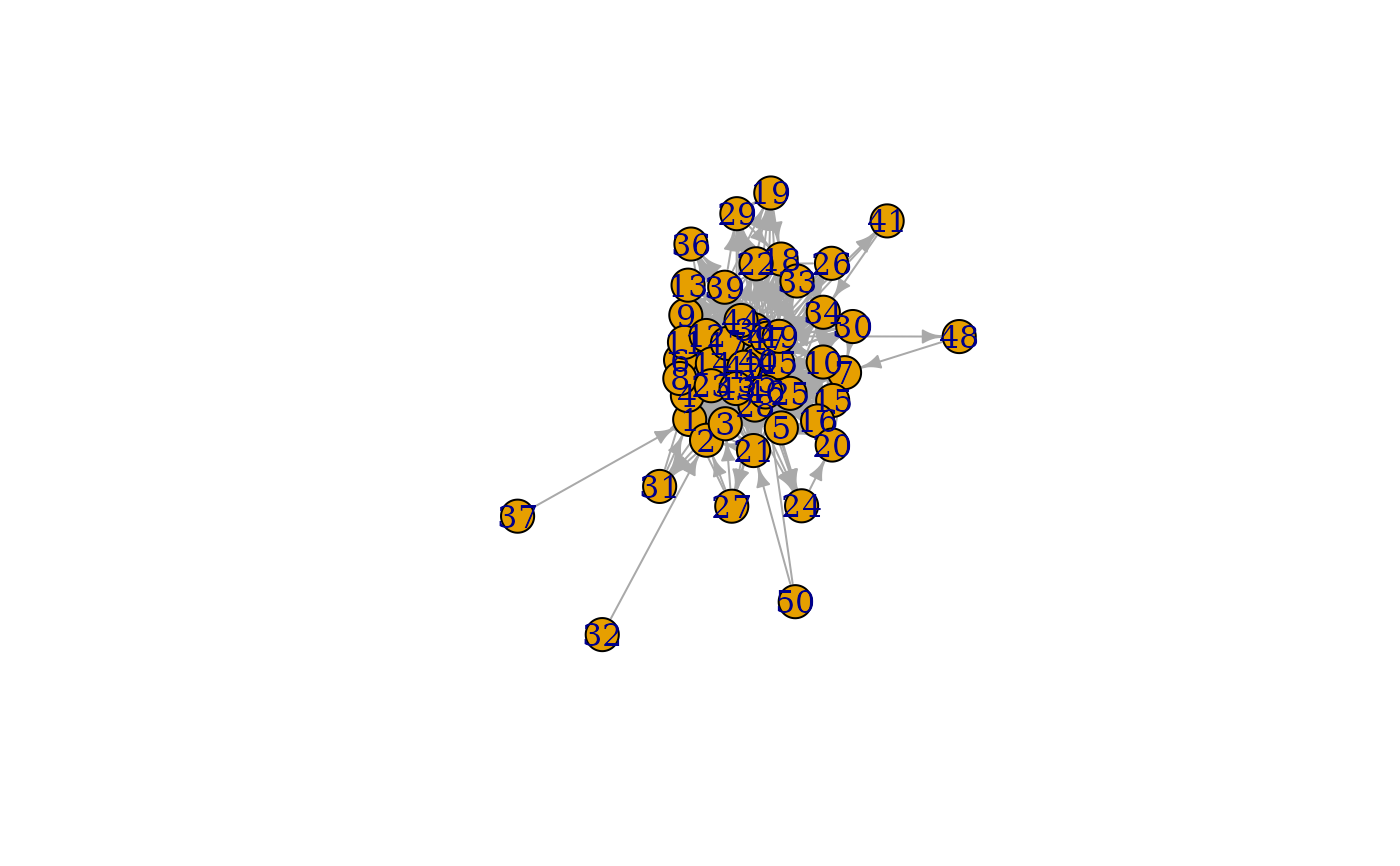This games create graphs through different types of evolutionary mechanisms (not necessarily in a biological sense). The nature of their algorithm is described in detail at the linked igraph documentation.

play_citation_age(
n,
growth = 1,
bins = n/7100,
p_pref = (1:(bins + 1))^-3,
directed = TRUE
)

play_forestfire(
n,
p_forward,
p_backward = p_forward,
growth = 1,
directed = TRUE
)

play_growing(n, growth = 1, directed = TRUE, citation = FALSE)

play_barabasi_albert(
n,
power,
growth = 1,
growth_dist = NULL,
use_out = FALSE,
appeal_zero = 1,
directed = TRUE,
method = "psumtree"
)

play_barabasi_albert_aging(
n,
power,
power_age,
growth = 1,
growth_dist = NULL,
bins = 300,
use_out = FALSE,
appeal_zero = 1,
appeal_zero_age = 0,
directed = TRUE,
coefficient = 1,
coefficient_age = 1,
window = NULL
)

## Arguments

n The number of nodes in the graph. The number of edges added at each iteration The number of aging bins The probability that an edge will be made to an age bin. Should the resulting graph be directed Forward and backward burning probability Should a citation graph be created The power of the preferential attachment The distribution of the number of added edges at each iteration Should outbound edges be used for calculating citation probability The appeal value for unconnected nodes The algorithm to use for graph creation. Either 'psumtree', 'psumtree-multiple', or 'bag' The aging exponent The appeal value of nodes without age The coefficient of the degree dependent part of attrictiveness The coefficient of the age dependent part of attrictiveness The aging window to take into account when calculating the preferential attraction

## Value

A tbl_graph object

## Functions

• play_citation_age: Create citation graphs based on a specific age link probability. See igraph::sample_last_cit()

• play_forestfire: Create graphs by simulating the spead of fire in a forest. See igraph::sample_forestfire()

• play_growing: Create graphs by adding a fixed number of edges at each iteration. See igraph::sample_growing()

• play_barabasi_albert: Create graphs based on the Barabasi-Alberts preferential attachment model. See igraph::sample_pa()

• play_barabasi_albert_aging: Create graphs based on the Barabasi-Alberts preferential attachment model, incoorporating node age preferrence. See igraph::sample_pa_age().

play_traits() and play_citation_type() for an evolutionary algorithm based on different node types
Other graph games: component_games, sampling_games, type_games
plot(play_forestfire(50, 0.5))